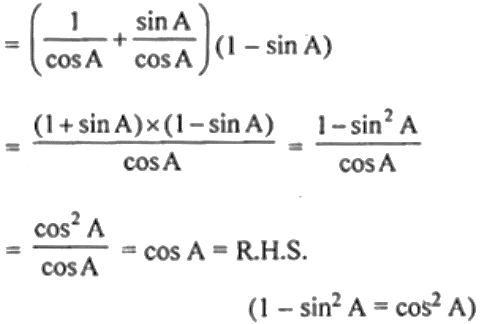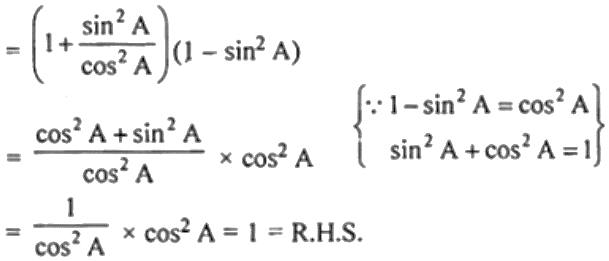Guru

# (i) (sec A + tan A) (1 – sin A) = cos A (ii) (1 + tan2 A) (1 – sin A) (1 + sin A) = 1

• 0

This is an important question taken from the Book- ML Aggarwal
Board- ICSE
Publication- Avichal
Chapter- Trigonometric Identities
Chapter number-1

We have to prove that
(i) (sec A + tan A) (1 – sin A) = cos A

(ii) (1 + tan2 A) (1 – sin A) (1 + sin A) = 1

ML Aggarwal, Icse board, chapter 18, ques no 12

Share

1. Solution:

Given,

(i) L.H.S = (sec A + tan A) (1 – sin A)(ii) L.H.S. = (1 + tan2 A) (1 – sin A) (1 + sin A)• 0